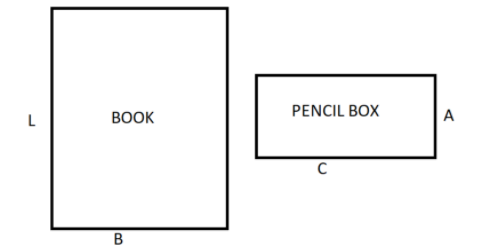QuestionAnswers

# Give any two examples for each from your daily life to find the area of the rectangle.Verified
129k+ views
Hint: In this particular question use the concept that a rectangle has opposite sides parallel to each other and the length of the adjacent sides are not equal so use these concepts to reach the solution of the question.

In our daily life these are lots of examples which resemble the shape of the rectangle.
As we all know that a rectangle has opposite sides parallel to each other and the length of the adjacent sides are not equal to each other but the length of the opposite sides are equal to each other and in a rectangle adjacent sides always make a 90 degrees.
For example: books, pencil box, doors, keyboard, laptops, mobile phones, etc.
So consider any two of the above examples such as books and pencil boxes.
The pictorial representation of the book and the pencil box are shown below.Let the length of the book and pencil box be L and A respectively, and the breadth of the book and the pencil box be B and C respectively as shown in the above figure.
Now as we know that the area of the rectangle is the multiplication of the length and the breadth.
So the area (${A_1}$) of the book is
$\Rightarrow {A_1} = L \times B$ Square units.
And the area (${A_2}$) of the pencil box is
$\Rightarrow {A_2} = A \times C$ Square units.
So this is the required answer.

Note: Whenever we face such types of questions the key concept we have to remember is that we always recall the definition of a rectangle which is stated above without knowing this we cannot choose the items from our daily life which resembles the shape of the rectangle.